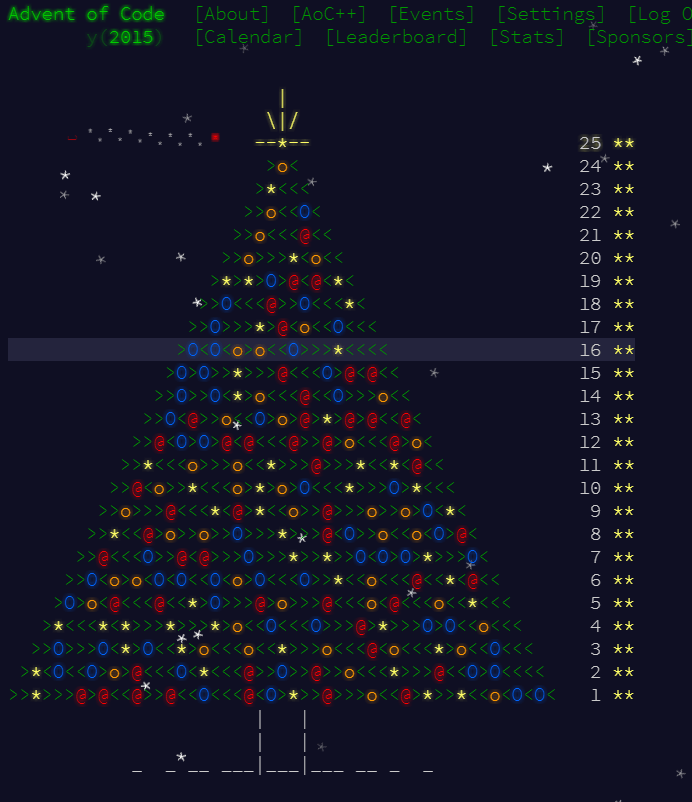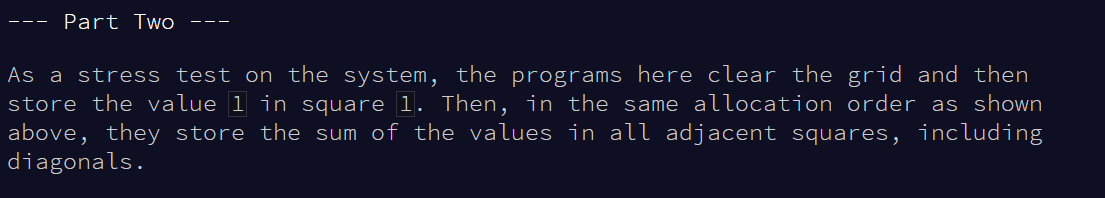# Advent of Code: Day 3

It’s day 3 of the Advent of Code, so day 3 of my set-based approach to solving the challenges.

The Challenge

The challenge today was the Spiral Memory challenge.The first challenge was, given a number, to determine the number of steps from the centre of the grid to that number. It’s pretty clear that this problem is not really suited to a set-based approach, but I did try to use it anyway.

The first thing I noticed was that the diagonal starting in the middle of the grid, and going down and right, was the squares of the odd numbers (1…9…25…). I decided to use this fact to determine which “spiral” my puzzle input could be found in. In my case, the answer was 515. This meant that my square would extend 257 steps in each direction from the starting block. I decided to work with the co-ordinates of each square, starting at (0,0).

```declare @puzzleinput bigint = 265149

declare @gridsize int,
@startvalue int

select top 1 @gridsize = Number,
@startvalue = POWER(LAG(Number,1,0) over (order by Number) ,2)
from Utility.dbo.TallyTable
where Number % 2 = 1
and Number - 1 < SQRT(@puzzleinput)
order by Number desc

declare @extreme int = (@gridsize - 1) / 2```

I created a table to store the co-ordinates of the number in the square. I then built only the outside spiral, by using my tally table again (see Day 1 for more info). The right hand side of the grid had a y-coordinate of 257, and starts with an x-coordinate of 257 and moves to -257. The other sides all follow a similar pattern, having one static coordinate, and one which moves. By starting with the square of the previous odd number (513), I could easily work out what position my puzzle input was found at. Calculating the distance to (0,0) was the pretty simple, it was just the absolute value of my x-coordinate plus the absolute value of the y-coordinate.

```declare @coordtable table (x int, y int, sqno int identity(1,1) )

-- insert the right hand side of the block

insert into @coordtable
select Number - @extreme - 1, @extreme
from Utility.dbo.TallyTable
where Number between 1 and @gridsize - 1
order by Number desc

-- insert the top of the block

insert into @coordtable
select -1 * @extreme , Number - @extreme - 1
from Utility.dbo.TallyTable
where Number between 1 and @gridsize - 1
order by Number desc

-- insert the left hand side of block

insert into @coordtable
select Number - @extreme, -1 * @extreme
from Utility.dbo.TallyTable
where Number between 1 and @gridsize - 1
order by Number asc

-- insert the bottom of block

insert into @coordtable
select @extreme, Number - @extreme
from Utility.dbo.TallyTable
where Number between 1 and @gridsize - 1
order by Number asc

SELECT abs(x) + abs(y) as Answer1
FROM @coordtable
where sqno + @startvalue = @puzzleinput```

So that worked pretty well, and it unlocked part 2 of the question.And… the question needed a totally different solution. Where the previous part only required 1 value, this part needed to know all the cells around it. So I would have to change my method entirely.

```declare @answer2 int

declare @x int = 0,
@y int = 0,
@dir char(1) = 'E'

declare @move table
(
dir char(1),
x int,
y int
)

set @extreme = 1

insert into @move
values
('N',0,-1),
('W',-1,0),
('S',0,1),
('E',1,0)```

I used a similar idea, but I couldn’t insert entire rows at once, I had to load each value individually so I could calculate the value of the cells around it. The only benefit that set-based thinking was that it was super easy to calculate the value of the blocks that were already “filled”.

```while @puzzleinpupt > (select max(val) from @coordtable2)
begin

if @x = @extreme and @y = @extreme set @extreme += 1
if @dir = 'E' and @x = @extreme set @dir = 'N'
if @dir = 'N' and @y = -1 * @extreme set @dir = 'W'
if @dir = 'W' and @x = -1 * @extreme set @dir = 'S'
if @dir = 'S' and @y = @extreme set @dir = 'E'

select @x = @x + x,
@y = @y + y
from @move
where dir = @dir

insert into @coordtable2
select @x, @y, sum(val)
from @coordtable2
where x between @x - 1 and @x + 1
and y between @y - 1 and @y + 1

end